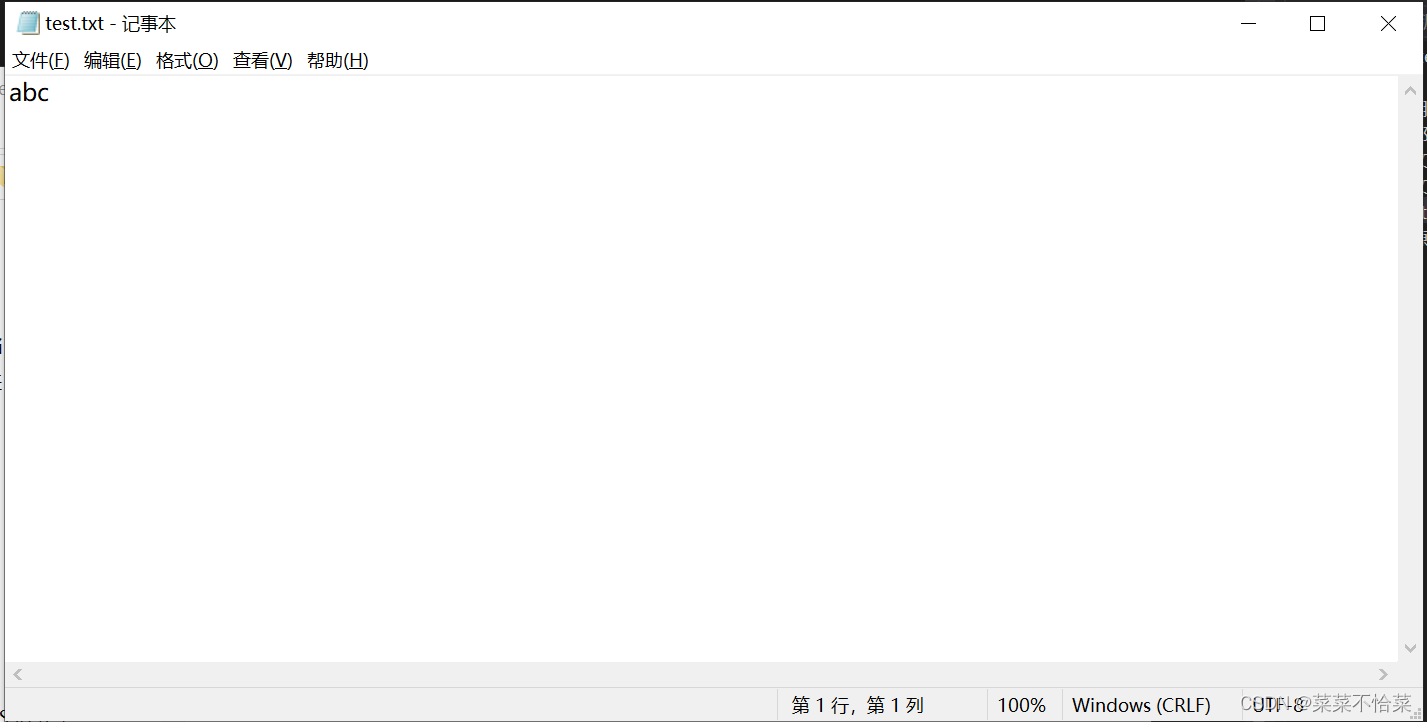﻿ C语言全面细致讲解文件操作_C 语言_脚本之家
C 语言# C语言全面细致讲解文件操作## 文件指针

FILE* pf;//文件指针变量

## 文件的打开和关闭

ANSIC 规定使用fopen函数来打开文件，fclose来关闭文件。

//打开文件

FILE * fopen ( const char * filename, const char * mode );

//关闭文件

int fclose ( FILE * stream );

```#include <stdio.h>
int main()
{
FILE* pf = fopen("test.txt", "w");//打开文件，以写的方式打开，文件名叫"test,txt"
if (pf == NULL)
{
perror("fopen");
return 1;
}
//文件操作
//关闭文件
fclose(pf);
pf = NULL;
return 0;
}```## 文件的顺序读写## 字符输入输出函数

fputc字符输出函数

int fputc ( int character, FILE * stream );//库函数中的定义

```#include <stdio.h>
int main()
{
FILE* pf = fopen("test.txt", "w");//打开文件名，以写的方式打开
if (pf == NULL)
{
perror("fopen");
return 1;
}
写文件
fputc('a', pf);//往文件里输出
fputc('b', pf);
fputc('c', pf);
//文件在被操作过程的时候其实是有一个位置信息的，在操作过程中时刻发生变化
关闭文件
fclose(pf);
pf = NULL;
return 0;
}
```fgetc（字符输入函数）

int fgetc ( FILE * stream );

```#include <stdio.h>
int main()
{
FILE* pf = fopen("test.txt", "r");//打开文件名，以读的方式打开
if (pf == NULL)
{
perror("fopen");
return 1;
}
//读文件
char ch=fgetc(pf);//这是我们不知道读到了什么可以打出来看看
printf("%c\n", ch);
ch = fgetc(pf);
printf("%c\n", ch);
ch = fgetc(pf);
printf("%c\n", ch);
//关闭文件
fclose(pf);
pf = NULL;
return 0;
}
``````#include <stdio.h>
int main()
{
{
return 1;
}
FILE* pfWrite = fopen("test2.txt", "w");
if (pfWrite == NULL)
{
return 1;
}
char ch = 0;
{
fputc(ch, pfWrite);
}
//关闭文件
fclose(pfWrite);
pfWrite = NULL;
return 0;
}
```## 字符串输入输出函数(fgets,fputs)

int fputs ( const char * str, FILE * stream );

```#include <stdio.h>
int main()
{
FILE* pf = fopen("test.txt", "w");//打开文件名，以写的方式打开
if (pf == NULL)
{
perror("fopen");
return 1;
}
//写数据
fputs("hello world\n",pf);\\不会自动换行，所以自己可以加上换行符
fputs("haha\n", pf);
fclose(pf);
pf = NULL;
return 0;
}
```char * fgets ( char * str, int num, FILE * stream );

```#include <stdio.h>
int main()
{
char input = { 0 };//将读到的数据存放进来
FILE* pf = fopen("test.txt", "r");//打开文件名，以写的方式打开
if (pf == NULL)
{
perror("fopen");
return 1;
}
//读数据
fgets(input, 20, pf);
printf("%s", input);
fgets(input, 20, pf);
printf("%s", input);
fclose(pf);
pf = NULL;
return 0;
}
```## 格式化输入输出函数(fscanf,fprintf)

int fprintf ( FILE * stream, const char * format, … );

```#include <stdio.h>
struct stu
{
char name;
int age;
float score;
};
int main()
{
struct stu s = { "zhangsan",20,66.5f };
FILE* pf = fopen("test.txt", "w");
if (pf == NULL)
{
perror("fopen");//如果打开失败，打印失败原因
return 1;
}
fprintf(pf, " %s %d %f", s.name, s.age, s.score);
fclose(pf);
pf = NULL;
return 0;
}
```

int fscanf ( FILE * stream, const char * format, … );

```#include <stdio.h>
struct stu
{
char name;
int age;
float score;
};
int main()
{
struct stu s = { 0 };
FILE* pf = fopen("test.txt", "r");
if (pf == NULL)
{
perror("fopen");
return 1;
}
fscanf(pf, "%s %d %f", s.name,&( s.age), &(s.score));
printf("%s %d %f", s.name, s.age, s.score);
fclose(pf);
pf = NULL;
return 0;
}
```

size_t fwrite ( const void * ptr, size_t size, size_t count, FILE * stream );

```#include <stdio.h>
struct stu
{
char name;
int age;
float score;
};
#include <stdio.h>
int main()
{
struct stu s = { "zhangsan",20,66.5f };
FILE* pf = fopen("test.txt", "wb");
if (pf == NULL)
{
perror("fopen file for writting");
return 1;
}
fwrite(&s,sizeof(struct stu),1,pf);
fclose(pf);
pf = NULL;
return 0;
}
```size_t fread ( void * ptr, size_t size, size_t count, FILE * stream );

```#include <stdio.h>
struct stu
{
char name;
int age;
float score;
};
#include <stdio.h>
int main()
{
struct stu s = {0 };
FILE* pf = fopen("test.txt", "rb");
if (pf == NULL)
{
perror("fopen file for writting");
return 1;
}
printf("%s %d %f", s.name, s.age, s.score);
fclose(pf);
pf = NULL;
return 0;
}
```## 文件的随机读写

### fseek

int fseek ( FILE * stream, long int offset, int origin );

long int offset是偏移量， int origin是偏移起始位置。

SEEK_SET：从起始位置开始偏移

SEEK_END：从末尾开始偏移

SEEK_CUR:从当前位置开始偏移

```#include <stdio.h>
int main()
{
FILE* pf = fopen("test.txt", "w");
if (pf == NULL)
{
perror("open");
return 1;
}
fputs("ABCDEF", pf);
fseek(pf,2, SEEK_SET);//从起始位置开始偏移
/*fseek(pf,-2, SEEK_CUR);*///从当前位置开始偏移
/*fseek(pf,-3, SEEK_END);*///从末尾开始偏移
fputc('G', pf);
fclose(pf);
pf = NULL;
return 0;
}```### ftell

long int ftell ( FILE * stream );

```#include <stdio.h>
int main()
{
FILE* pf = fopen("test.txt", "w");
if (pf == NULL)
{
perror("open");
return 1;
}
fputs("ABCDEF", pf);
fseek(pf,2, SEEK_SET);//这时候指针指向了从起始位置开始偏移，偏移量为2的位置上的C
int ret = ftell(pf);//因为此时指针指向C，它相当于起始位置偏移量为2
printf("ret = %d\n", ret);
fclose(pf);
pf = NULL;
return 0;
}
```## 文件读取结束的判定

feof这个函数要牢记：在文件读取过程中，不能用feof函数的返回值直接用来判断文件的是否结束。

1.文本文件读取是否结束，判断返回值是否为 EOF （ fgetc ），或者 NULL （ fgets ）

fgetc 判断是否为 EOF .

fgets 判断返回值是否为 NULL .

2.二进制文件的读取结束判断，判断返回值是否小于实际要读的个数。

```#include <stdio.h>
#include <stdlib.h>
int main(void) {
int c; // 注意：int，非char，要求处理EOF
FILE* fp = fopen("test.txt", "r");
if(!fp) {
perror("File opening failed");
return EXIT_FAILURE;
}
//fgetc 当读取失败的时候或者遇到文件结束的时候，都会返回EOF
while ((c = fgetc(fp)) != EOF) // 标准C I/O读取文件循环
{
putchar(c);
}
//判断是什么原因结束的
if (ferror(fp))
else if (feof(fp))
puts("End of file reached successfully");
fclose(fp);
}
```

```#include <stdio.h>
enum { SIZE = 5 };
int main(void) {
double a[SIZE] = {1.,2.,3.,4.,5.};
FILE *fp = fopen("test.bin", "wb"); // 必须用二进制模式
fwrite(a, sizeof *a, SIZE, fp); // 写 double 的数组
fclose(fp);
double b[SIZE];
fp = fopen("test.bin","rb");
size_t ret_code = fread(b, sizeof *b, SIZE, fp); // 读 double 的数组
if(ret_code == SIZE)
{
for(int n = 0; n < SIZE; ++n) printf("%f ", b[n]);
putchar('\n');
}
else
{
// error handling
if (feof(fp))
printf("Error reading test.bin: unexpected end of file\n");
else if (ferror(fp))
{